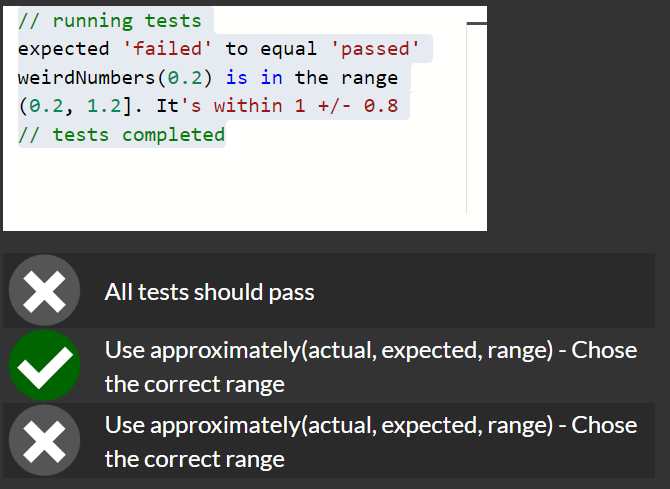# Quality Assurance and Testing with Chai - Test if a Value Falls within a Specific Range

That’s the correct solution but why is that?

``````    test('#approximately', function() {
assert.approximately(weirdNumbers(0.5) , 1, /*edit this*/0.5 );
assert.approximately(weirdNumbers(0.2) , 1, /*edit this*/ 0.8 );
});
});
``````

Am I not supposed to check the range of the values that can be returned by the weirdNumbers function?

delta=0.5
`1+0.5 - random number (0-1.0)`
The range should be ` [0.5,1.5]`
`1+0.5-1=0.5 1+0.5-0.0=1.5`

delta=0.2
`1+0.2 - random number (0-1.0)`
`[0.2,1.2]`

``````/*
function weirdNumbers(delta) {
return (1 + delta - Math.random());
}

*/

/** 10 - .approximately **/
// .approximately(actual, expected, range, [message])
// actual = expected +/- range
// Choose the minimum range (3rd parameter) to make the test always pass
// it should be less than 1
test('#approximately', function() {
assert.approximately(weirdNumbers(0.5) , 1.5, /*edit this*/0.5 );
assert.approximately(weirdNumbers(0.2) , 1.2, /*edit this*/ 0.2 );
});
});
``````

I am probably missing some math behind it

``````  /*
.approximately(actual, expected, range, [message])

actual = expected +/- range

*/
``````The only thing I notice is that it could be `1-delta`
ex: `1-0.2=0.8 or 1-0.5=0.5`

If you found this message - your search is over.

To understand what you need to do, GET your_url_on_glitch/ _api/get-tests?type=unit&n=9 with the help of Postman

Your last comment is the key. Since the maximum value that the random number could be is 1, you need to add enough to the delta value to bring the total back up to 1, (1 is the expected value), in case the random number turns out to be 1 and 1 is subtracted from delta + 1.

1 Like

What does delta mean here? It’s not ‘rate of change’, right? Is this definition – not sure what the def is – unique to programming? I sort of get this answer, but it surprised me as well.